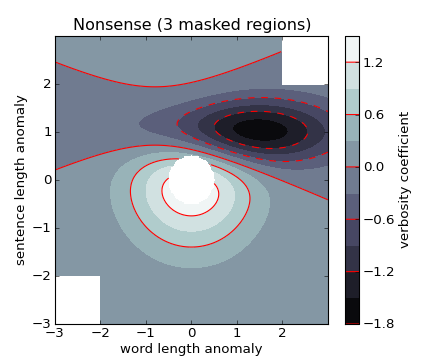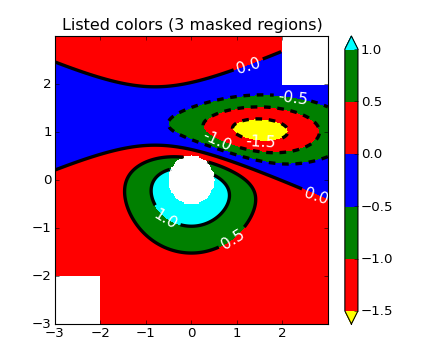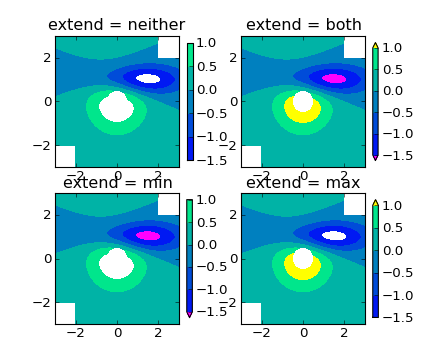pylab_examples example code: contourf_demo.py¶(png, hires.png, pdf)(png, hires.png, pdf)(png, hires.png, pdf)

#!/usr/bin/env python
import numpy as np
import matplotlib.pyplot as plt

origin = 'lower'
#origin = 'upper'

delta = 0.025

x = y = np.arange(-3.0, 3.01, delta)
X, Y = np.meshgrid(x, y)
Z1 = plt.mlab.bivariate_normal(X, Y, 1.0, 1.0, 0.0, 0.0)
Z2 = plt.mlab.bivariate_normal(X, Y, 1.5, 0.5, 1, 1)
Z = 10 * (Z1 - Z2)

nr, nc = Z.shape

# put NaNs in one corner:
Z[-nr//6:, -nc//6:] = np.nan
# contourf will convert these to masked

Z = np.ma.array(Z)

# mask a circle in the middle:
interior = np.sqrt((X**2) + (Y**2)) < 0.5

# We are using automatic selection of contour levels;
# this is usually not such a good idea, because they don't
# occur on nice boundaries, but we do it here for purposes
# of illustration.
CS = plt.contourf(X, Y, Z, 10, # [-1, -0.1, 0, 0.1],
#alpha=0.5,
cmap=plt.cm.bone,
origin=origin)

# Note that in the following, we explicitly pass in a subset of
# the contour levels used for the filled contours.  Alternatively,
# We could pass in additional levels to provide extra resolution,
# or leave out the levels kwarg to use all of the original levels.

CS2 = plt.contour(CS, levels=CS.levels[::2],
colors = 'r',
origin=origin,
hold='on')

plt.xlabel('word length anomaly')
plt.ylabel('sentence length anomaly')

# Make a colorbar for the ContourSet returned by the contourf call.
cbar = plt.colorbar(CS)
cbar.ax.set_ylabel('verbosity coefficient')
# Add the contour line levels to the colorbar

plt.figure()

# Now make a contour plot with the levels specified,
# and with the colormap generated automatically from a list
# of colors.
levels = [-1.5, -1, -0.5, 0, 0.5, 1]
CS3 = plt.contourf(X, Y, Z, levels,
colors = ('r', 'g', 'b'),
origin=origin,
extend='both')
# Our data range extends outside the range of levels; make
# data below the lowest contour level yellow, and above the
# highest level cyan:
CS3.cmap.set_under('yellow')
CS3.cmap.set_over('cyan')

CS4 = plt.contour(X, Y, Z, levels,
colors = ('k',),
linewidths = (3,),
origin = origin)
plt.clabel(CS4, fmt = '%2.1f', colors = 'w', fontsize=14)

# Notice that the colorbar command gets all the information it
# needs from the ContourSet object, CS3.
plt.colorbar(CS3)

# Illustrate all 4 possible "extend" settings:
extends = ["neither", "both", "min", "max"]
cmap = plt.cm.get_cmap("winter")
cmap.set_under("magenta")
cmap.set_over("yellow")
# Note: contouring simply excludes masked or nan regions, so
# instead of using the "bad" colormap value for them, it draws
# nothing at all in them.  Therefore the following would have
# no effect: